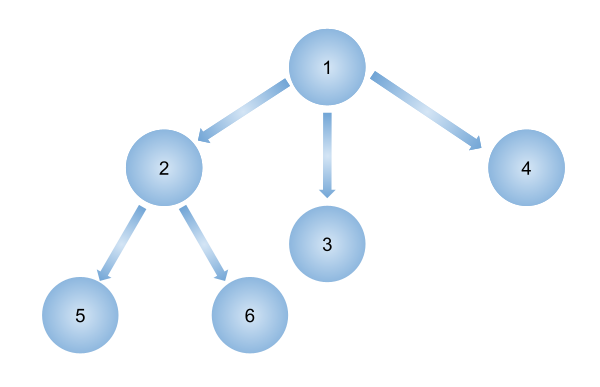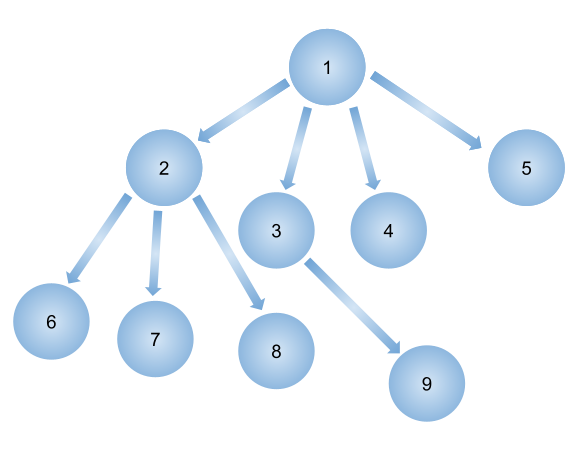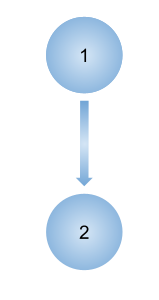0

# N-ary tree Level Order Traversal

Difficulty: EASY
Avg. time to solve
20 min
Success Rate
80%

Problem Statement
Suggest Edit

#### You are given an N-ary tree where every node has at most ‘N’ child nodes. You need to find the level order traversal of this tree

##### Note:
`````` Note that the level order traversal must not contain any null values, simply return the tree in level order.
``````
##### Input Format:
``````The first line contains an integer 'T' which denotes the number of test cases or queries to be run. Then the test cases are as follows.

The first line of each test case contains elements of the N-ary tree in the level order form. The line consists of values of nodes separated by a single space. In case a node is changed, we take -1. To get next node
The first not-null node(of the previous level) is treated as the parent of the first node of the current level. The second not-null node (of the previous level) is treated as the parent node for the next nodes of the current level and so on.
The input ends when all nodes at the last level are null(-1).
``````
##### Note :
``````The above format was just to provide clarity on how the input is formed for a given tree.
The sequence will be put together in a single line separated by a single space. Hence, for the below-depicted tree, the input will be given as:
1 2 3 4 -1 5 6 -1 -1 -1 -1 -1
``````##### Output Format:
``````For each test case, you need to print the level order traversal of the tree.

Print the output of each test case in a separate line.
``````
##### Note:
``````You don’t need to print anything; It has already been taken care of.
``````
##### Constraints:
``````1 <= T <= 10
1 <=  K <= 10000

where ‘T’ is the number of test cases, ‘K’ denotes the number of nodes in the N-ary tree.

Time limit: 1 sec
``````
##### Sample Input 1:
``````1
1 2 3 4 -1 5 6 -1 -1 -1 -1 -1
``````
##### Sample Output 1:
``````1 2 3 4 5 6
``````
##### Explanation of sample input 1:
``````In the first test case,
Given tree:
````````````The level order traversal of the above tree is: 1 2 3 4 5 6
``````
##### Sample Input 2:
``````2
1 2 3 4 5 -1 6 7 8 -1 9 -1 -1 -1 -1 -1 -1 -1
1 2 -1
``````
##### Sample Output 2:
``````1 2 3 4 5 6 7 8 9
1 2
``````
##### Explanation of sample input 2:
``````In the first test case,
Given tree:
````````````The level order traversal of the above tree is: 1 2 3 4 5 6 7 8 9

In the second test case,
Given tree:
````````````The level order traversal of the above tree is: 1 2
``````Want to solve this problem? Login now to get access to solve the problems